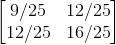# Finding state vectors for pure states

• Pouyan

## Homework Statement

Is the following matrix a state operator ? and if it is a state operator is it a pure state ? and if it is so then find the state vectors for the pure state.If you don't see image here is the matrix which is 2X2 in MATLAB code:
[9/25 12/25; 12/25 16/25]

## Homework Equations

To be a state operator, if we have a operator ρ we know :

Tr(ρ)=1
<u|ρ|u> >= 0 for all vectors |u>
and these means :
the sum of eigenvalues must be 1 and eigenvalues must be greater or equal to zero

For pure state what do I know are these:
ρ=|ψ><ψ| where |ψ> is the unit-normed vector called state vector.
The average value of an observable R in this pure state is:
<R> = Tr(|ψ><ψ|R) = <ψ|R|ψ>
The other condition is :
ρ2=ρ (which is possible for 1 or 0 but the sum of eigenvalues must be 1)
The third condition is :
Tr(ρ2)=1

## The Attempt at a Solution

This matrix has eigenvalues 1 and 0. And this means it is a state operator. In my solution I do see that this matrix is a pure state and it has the vector state : (3/5 4/5). But I don't know how I can use conditions for pure state to see that if a matrix or an operator is a pure state and I can not either get the state vectors.

What do I know is that :
WWith eigenvalue 1 we get vector (-(4/3) 1). I do see that (3/5 4/5) is the norm of the diagonal of the matrix, [9/25 12/25; 12/25 16/25], that is in the first place in the matrix we have 9/25 and √(9/25)= 3/5.
In the last place of this matrix we have 16/25 and √(16/25) = 4/5
ofcourse 16/25 + 9/25 =1
But is that correct to think so ?

#### Attachments

Right. A projection operator (onto a subspace) when divided by the dimension of the subspace becomes a valid density operator (sometimes referred to as a "crisp" state). So it will have eigen-values of 0 for vectors of the null space and 1/n for vectors in the subspace of dimension n. If the projection operator is already a density operator, and thus has both eigenvaue 1 and trace 1, it must project onto a 1 dimensional subspace and thus represent a sharp state.

It is ##\lvert \psi \rangle \langle \psi\rvert## for the eigen-vector ##\lvert \psi \rangle##.